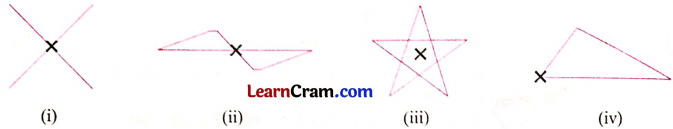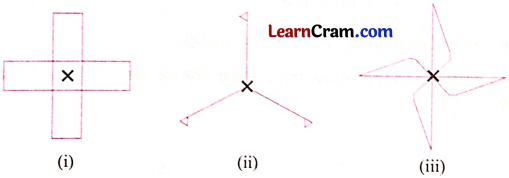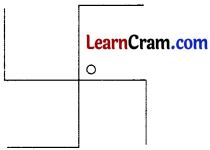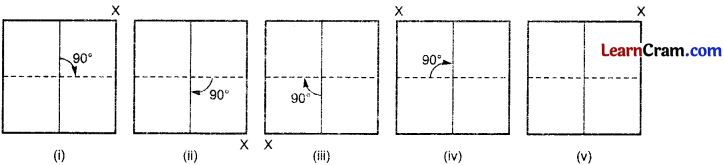# DAV Class 8 Maths Chapter 16 Worksheet 1 Solutions

The DAV Class 8 Maths Solution and DAV Class 8 Maths Chapter 16 Worksheet 1 Solutions of Rotational Symmetry offer comprehensive answers to textbook questions.

## DAV Class 8 Maths Ch 16 WS 1 Solutions

Question 1.
Which of the following figures have rotational symmetry about a marked point?Solution:

Fig. (i), (ii) and (iii) have rotational symmetry about the marked point.

Question 2.
Give the order of rotational symmetry of the given figures at the marked point:Solution:
Figure (i) has 4 orders of rotation.
Figure (ii) has 3 orders of rotation.
Figure (iii) has 4 orders of rotation.Question 3.
Which of the following figures have rotational symmetry of order more than 1?Solution:
Figure (i) has the order of rotation more than 1.
Figure (iii) has the order of rotation more than 1.
Figure (iv) has the order of rotation more than 1.

Question 4.
Find the angle of rotation and order of rotation for the given figure.Solution:
If the given figure is rotated about the fixed point O, there are four positions in which it looks exactly the same.
So, the given figure has rotational symmetry of order 4.
Angle of rotation of the given figure = $$\frac{360^{\circ}}{\text { Order of symmetry }}$$
= $$\frac{360^{\circ}}{4}$$ = 90°.### DAV Class 8 Maths Chapter 16 Worksheet 1 Notes

• Rotation takes place clockwise or anti-clockwise about a point known as centre of rotation.
• The angle through which the rotation takes place is called angle of rotation.
• Full rotation means turning through 360°.
• Half rotation means turning through 180°.
• Quarter rotation means turning through 90°.
• If an object attains the same position after taking many rotations, then the object has rotational symmetry.
• In a complete rotation, the number of rotations for which the object looks exactly the same, then it is called the order of rotational symmetry.Example 1.
In a complete rotation of a square, draw the four positions and answer the following:
(i) Centre of rotation
(ii) Angle of rotation
(iii) Direction of rotation
(iv) Order of rotation.
Solution:(i) Centre of rotation is the centre of the square
(ii) Angle of rotation is 90°.
(iii) Direction of rotation is clock-wise
(iv) Order of rotation is 4.